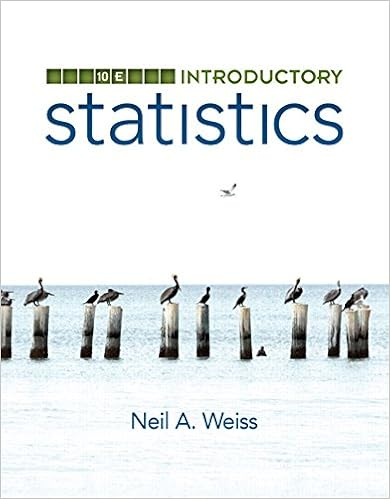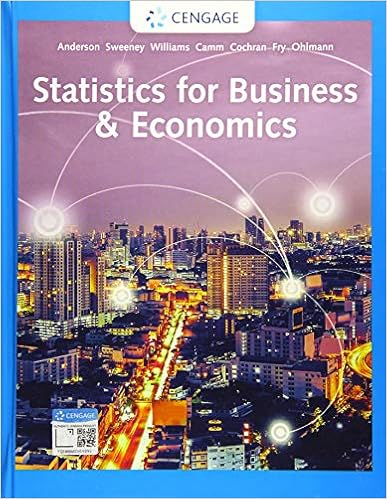# Appropriate where the analyst is completely uncertain

• Test Prep
• 312
• 80% (5) 4 out of 5 people found this document helpful

This preview shows page 82 - 89 out of 312 pages.

Appropriate where the analyst is completely uncertainabout a given cost, except that it must be between somespecified minimum and maximum values.Every valuebetween the minimum and the maximum has equalprobability of occurring.UniformTriangularProbably the most widely used distribution. Requiresminimum, maximum anda most likely value to beknown.The most likely represents the mode of thetriangle (its peak), and in pricing, would typically be thevalue used in the government objective.DiscreteUsed where discrete (distinct) events or outcomes existand the means are available to objectively or subjectivelystate the likelihood of each outcome occurring. The sum ofthe probabilities of the outcomes equals one.DISTRIBUTIONS82
##### We have textbook solutions for you!The document you are viewing contains questions related to this textbook.
Chapter 4 / Exercise 50
Elementary and Intermediate Algebra: Algebra Within Reach
LarsonExpert VerifiedBrowse all Textbook Solutions
Used when outcomes are equally likely to occur on eitherside of the average value; symmetric and continuous,unbounded, allowing for negative values. Need to be ableto determine the mean and standard deviation.NormalBeta (right skew) Beta (normal) Beta (left skew)Similar to the normal distribution, but does not allow for negative cost,this continuous distribution can be symmetric or skewed. The PERTbeta allows the user to specify minimum and maximum values, andthe shape parameters.DISTRIBUTIONS83
SYMMETRIC APPROXIMATION3.Calculate the mean and variance of each distribution84
SYMMETRIC APPROXIMATION4.Estimate the mean, variance, & SD for the total cost85
86CORRELATION – HOW COSTS ARE RELATEDIn our previous example we treated the elements of cost asif they wereindependentof each other.In other words,the cost of the Transmitter had no bearing on the cost ofthe Processor or Power Supply.But what if it did?What if the range on the Transmitter hada bearing on the demands on the Processor and theamount of power required by the Power Supply.In that case we would say that these components (andtheir costs) werecorrelated(i.e. a change in one wouldresult in a change in the other).
87CORRELATION – THE BOTTOM LINEIn order to understand how correlation affects the bottomline let’s take the example of direct manufacturing laborand manufacturing overhead.Since overhead is a function of the direct costs, we wouldsay that they are correlated.If there was an increase inour estimate of direct labor, we would also increase ourestimate of the overhead.Not doing so would understatethe total cost.Likewise, if our objective for direct labor decreased, thenwe would also decrease our estimate of overhead, andconsequently reduce the total cost.
88CORRELATION – THE BOTTOM LINEYou can see that if we treated the direct and indirect costsas independent, we would be understating both the lowerand upper bounds of the total cost. Or put another way,understating the variation in the total cost.

Course Hero member to access this document

Course Hero member to access this document

End of preview. Want to read all 312 pages?

Course Hero member to access this document

Term
Spring
Professor
N/A
Tags
Solicitation
##### We have textbook solutions for you!
The document you are viewing contains questions related to this textbook.The document you are viewing contains questions related to this textbook.
Chapter 4 / Exercise 50
Elementary and Intermediate Algebra: Algebra Within Reach
LarsonExpert Verified
•••